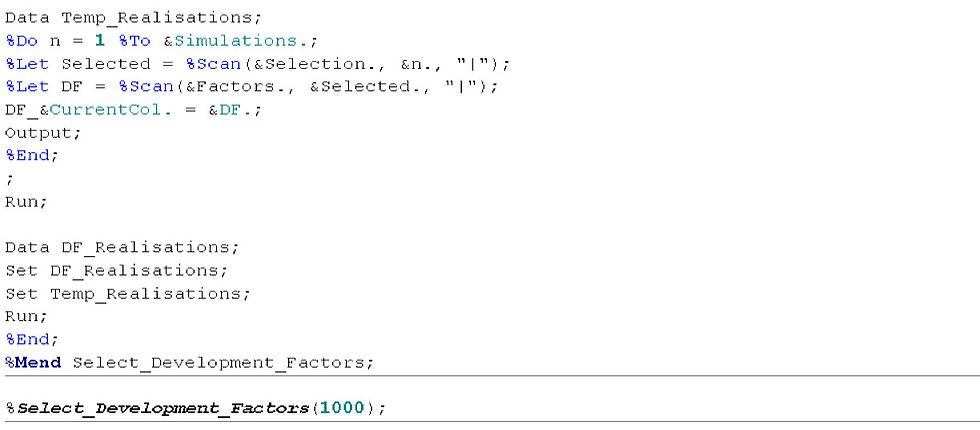top of page
Search

# Chain Ladder Simulations in Base SAS

In my last post I showed how SAS can be a tool in actuarial reserving. Particularly how to develop a loss triangle by using the volume weighted development factors. In this post I will extend this by showing a method for obtaining a distribution of possible reserves. This will be using a bootstrapping method of directly sampling the development factors.

I have referred in the code to a paid triangle but the method is the same with an incurred or any other type of triangle. As ever this post is a demonstration of what can be done in SAS. It is not intended as a guide to reserving or a recommendation of one particular reserving method over another.

For this post I am going to make an example monthly, monthly triangle with 60 rows and 60 columns. This is using code similar to my last post but I have changed the function slightly to make a triangle which mimics the behavior of a longer tail class of business with more volatility. I have also suppressed the log. This is because the macros are going to run repeatedly and I don’t want the log filled with unnecessary information.Next I obtain the development factor for each origin and development month.So I now have a triangle of observed development factors. I want to create from this triangle many possible development scenarios. I will do this by sampling one development factor from each development month to create each scenario. So each scenario has an observed development factor from the first column, one from the second, one from the third and so on. For the first column there are 59 possible factors. For the second there are 58 possible factors and so on.

The dataset DF_Realisations will have in it a line for each scenario and a column for each development month. In this column is the row number of the development factor from the triangle that is used in this scenario.

Using DF_Realisations a new dataset is created called Temp_Realisations. In this dataset is the development factors for each development month of each scenario.Finally I tidy the figures with the round command and output a frequency chart.For me 1,000 simulations took about 5 minute to run and output the results. Below I have pasted a chart of a frequency plot using 10,000 scenarios. In my next post I will demonstrate a way of using SAS to smooth the development factors using common statistical distributions and add a tail factor.